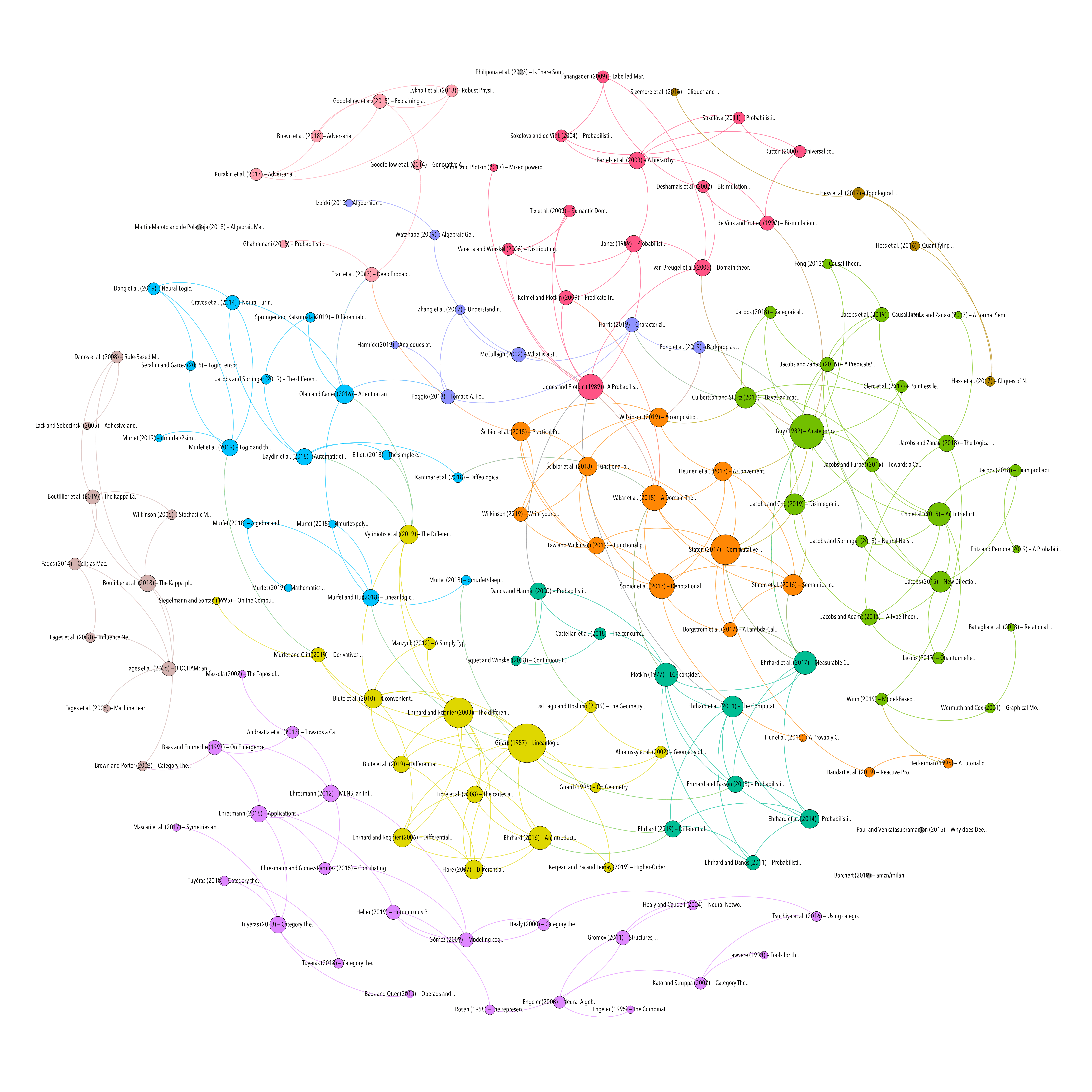A Convenient Category for Higher-Order Probability Theory

Resource type
Authors/contributors
Title
A Convenient Category for Higher-Order Probability Theory
Abstract
Higher-order probabilistic programming languages allow programmers to write sophisticated models in machine learning and statistics in a succinct and structured way, but step outside the standard measure-theoretic formalization of probability theory. Programs may use both higher-order functions and continuous distributions, or even define a probability distribution on functions. But standard probability theory does not handle higher-order functions well: the category of measurable spaces is not cartesian closed. Here we introduce quasi-Borel spaces. We show that these spaces: form a new formalization of probability theory replacing measurable spaces; form a cartesian closed category and so support higher-order functions; form a well-pointed category and so support good proof principles for equational reasoning; and support continuous probability distributions. We demonstrate the use of quasi-Borel spaces for higher-order functions and probability by: showing that a well-known construction of probability theory involving random functions gains a cleaner expression; and generalizing de Finetti's theorem, that is a crucial theorem in probability theory, to quasi-Borel spaces.
Publication
arXiv:1701.02547 [cs, math]
Date
2017-01-10
Accessed
2019-10-10T11:48:09Z
Library Catalog
Extra
arXiv: 1701.02547
Citation
Heunen, C., Kammar, O., Staton, S., & Yang, H. (2017). A Convenient Category for Higher-Order Probability Theory. ArXiv:1701.02547 [Cs, Math]. Retrieved from http://arxiv.org/abs/1701.02547
PROBABILITY & STATISTICS
Processing time: 0.01 secondsGraph of references (from Zotero to Gephi via Zotnet with this script)